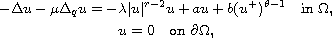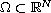Electron. J. Diff. Equ., Vol. 2014 (2014), No. 170, pp. 1-13.

### Nonlinear elliptic problem of 2-q-Laplacian type with asymmetric nonlinearities Dandan Yang, Chuanzhi Bai

Abstract:
In this article, we study the nonlinear elliptic problem of--Laplacian typewhereis a bounded domain. For a is between two eigenvalues, we show the existence of three nontrivial solutions.

Submitted July 9, 2014. Published August 11, 2014.
Math Subject Classifications: 35J60, 35B38.
Key Words: Quasilinear elliptic equations with q-Laplacian; critical exponent; asymmetric nonlinearity; weak solution.

Show me the PDF file (263 KB), TEX file, and other files for this article.Dandan Yang School of Mathematical Science, Huaiyin Normal University Huaian, Jiangsu 223300, China email: ydd423@sohu.com Chuanzhi Bai School of Mathematical Science, Huaiyin Normal University Huaian, Jiangsu 223300, China email: czbai@hytc.edu.cn